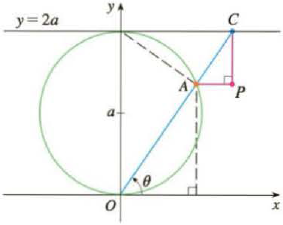Chapter 10.1, Problem 43E

Chapter
Section
Textbook Problem

# A curve, called a witch of Maria Agnesi, consists of all possible positions of the point P in the figure. Show that parametric equations for this curve can be written as x = 2 a cot θ y = 2 a sin 2 θ Sketch the curve.To determine

To find: The parametric equation for the curve given as x=2acotθ and y=2asin2θ .

Explanation

Given data:

The curve MariaAgnesi is shown in below figure1.

Calculation:

The horizontal distance of point C is x .

xOC=cosθ (1)

The vertical distance of point A is y .

yOA=sinθ (2)

The sum of all angles forming triangle OAD will be equal to 180 .

φ+θ=90φ=90θφ+90+α=18090θ+90+α=180α=θ

OA2a=sinθOA=sinθ2a

Substitute (sinθ2a) for OA in equation (2),

yOA=sinθ

### Still sussing out bartleby?

Check out a sample textbook solution.

See a sample solution

#### The Solution to Your Study Problems

Bartleby provides explanations to thousands of textbook problems written by our experts, many with advanced degrees!

Get Started

#### Find dy/dx by implicit differentiation. 15. ex/y =x y

Single Variable Calculus: Early Transcendentals, Volume I

#### In Problems 25-38, find y'. 37.

Mathematical Applications for the Management, Life, and Social Sciences

#### ∫ sin 3x cos 6x dx =

Study Guide for Stewart's Single Variable Calculus: Early Transcendentals, 8th

#### Evaluate each expression. (50)

College Algebra (MindTap Course List)